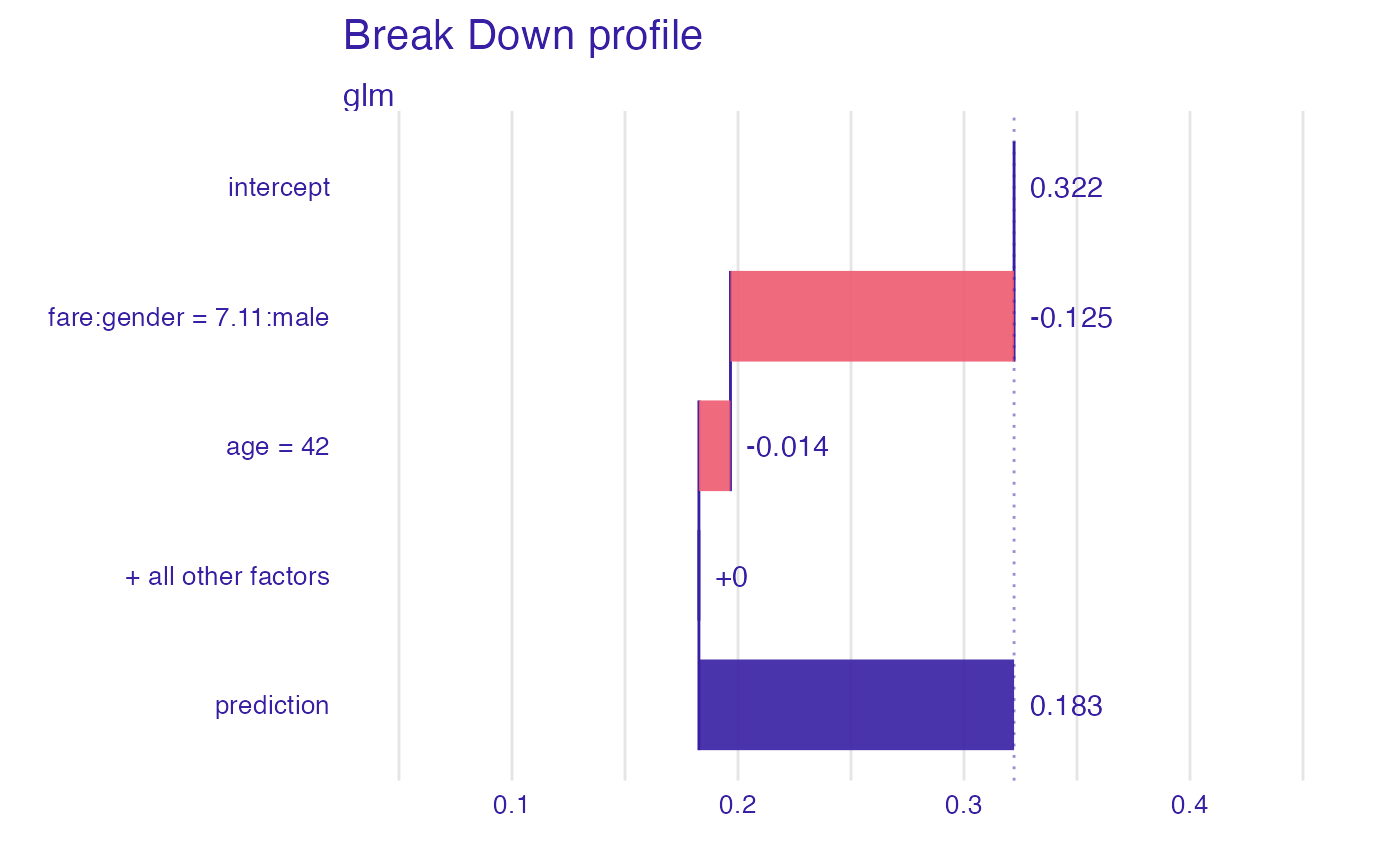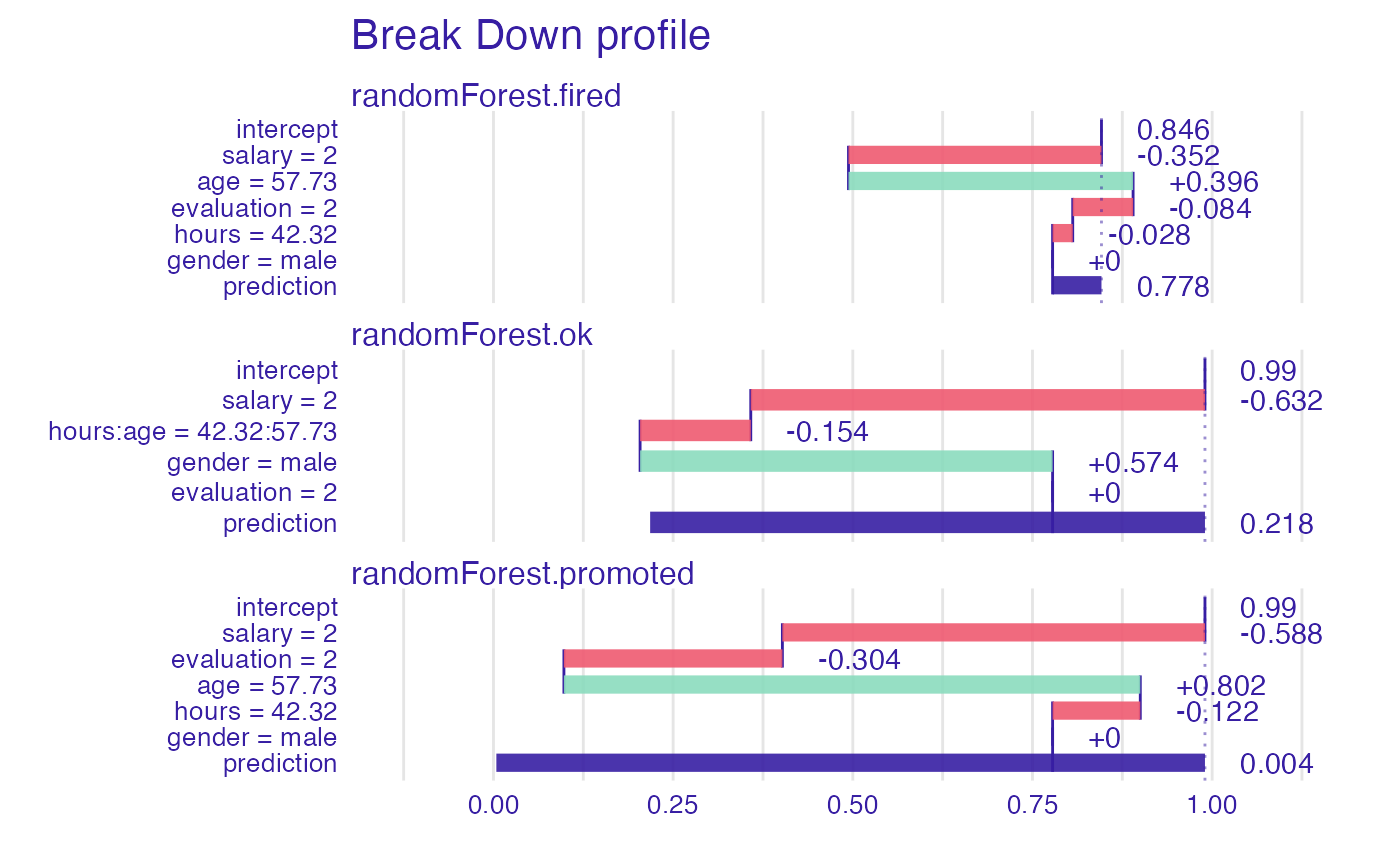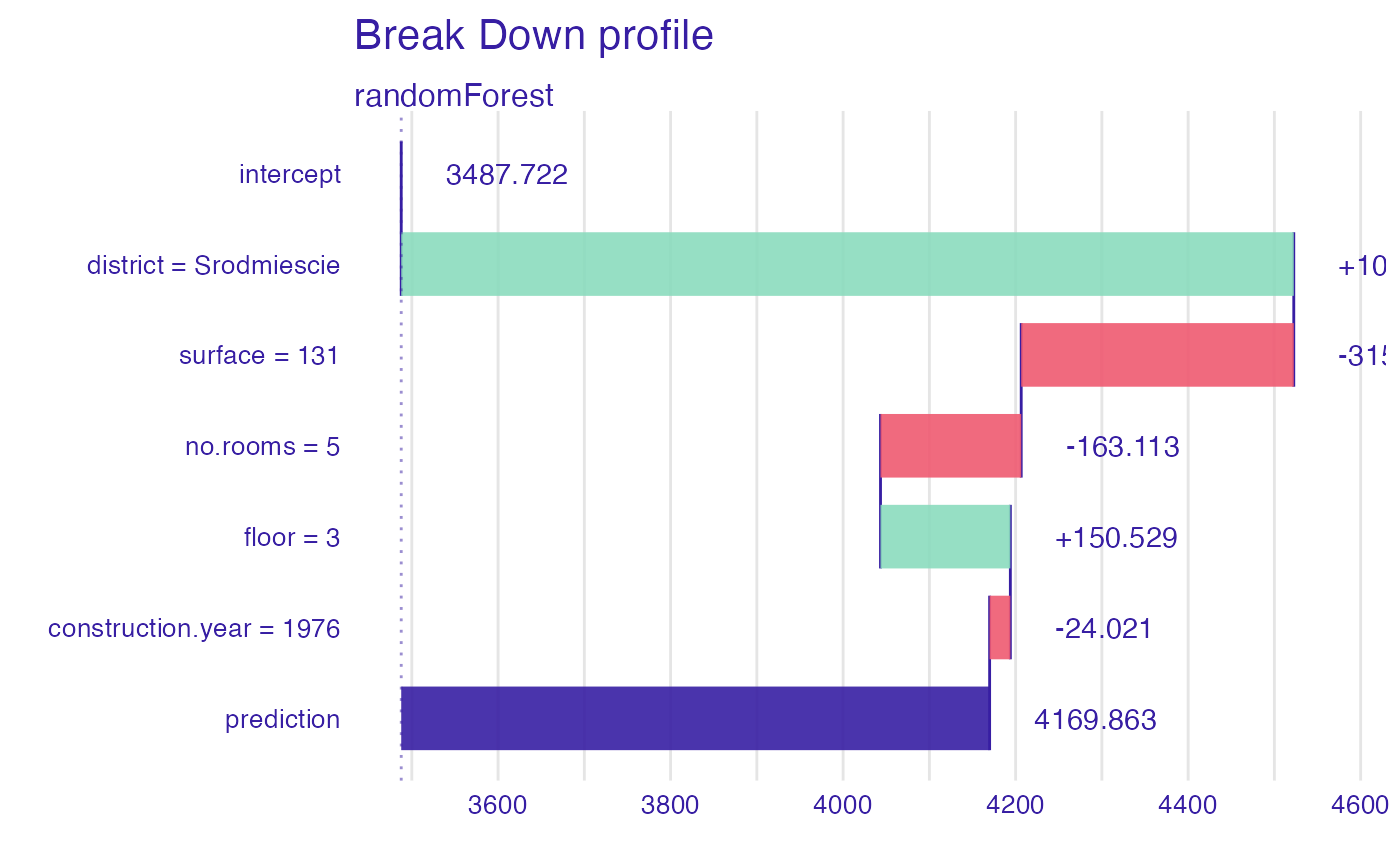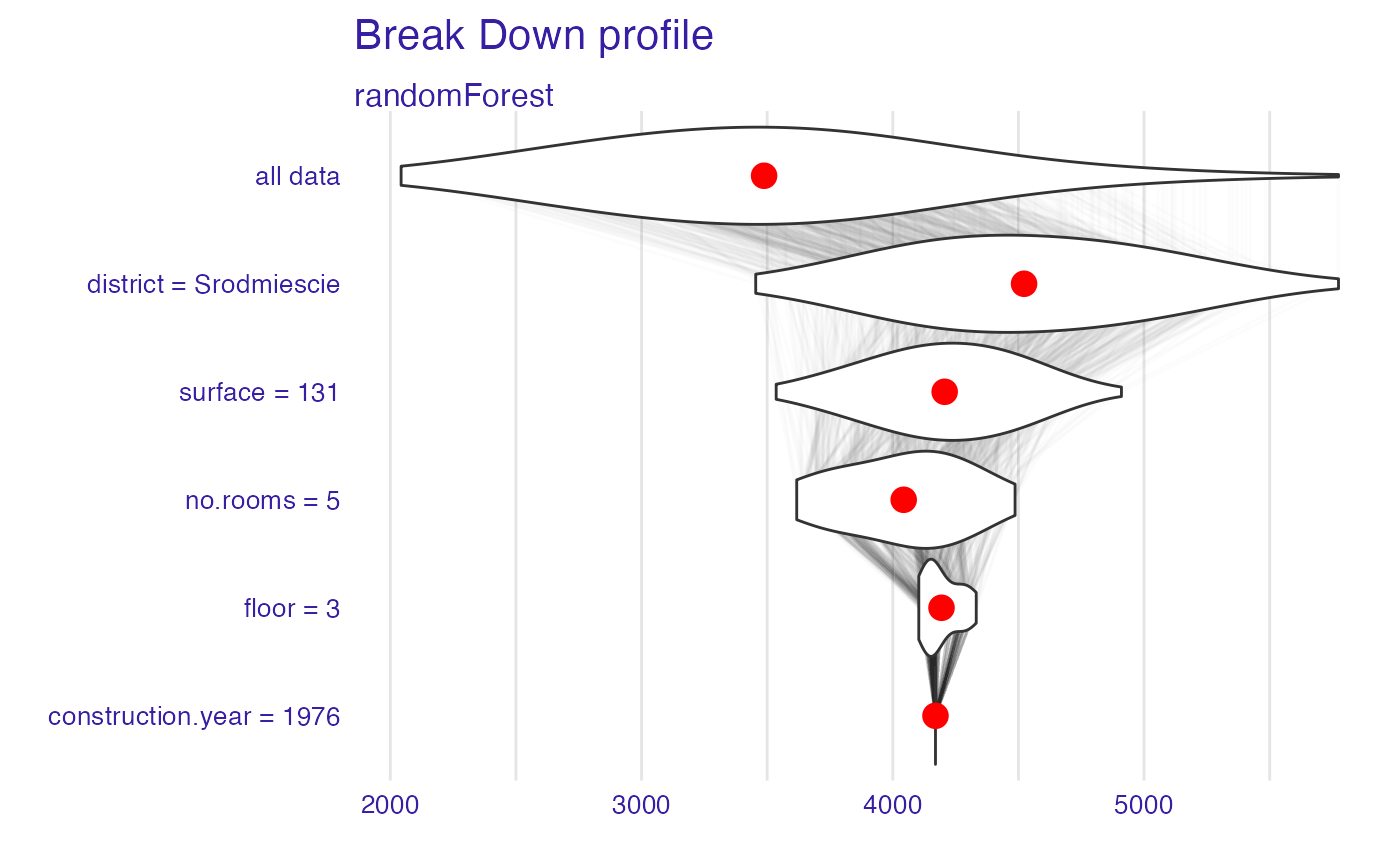This function implements decomposition of model predictions with identification of interactions. The complexity of this function is O(2*p) for additive models and O(2*p^2) for interactions. This function works in a similar way to step-up and step-down greedy approximations in function break_down(). The main difference is that in the first step the order of variables and interactions is determined. And in the second step the impact is calculated.

local_interactions(x, ...)

# S3 method for explainer
local_interactions(x, new_observation, keep_distributions = FALSE, ...)

# S3 method for default
local_interactions(
x,
data,
predict_function = predict,
new_observation,
label = class(x),
keep_distributions = FALSE,
order = NULL,
interaction_preference = 1,
...
)

## Arguments

x an explainer created with function explain or a model. other parameters. a new observation with columns that correspond to variables used in the model. if TRUE, then the distribution of partial predictions is stored in addition to the average. validation dataset, will be extracted from x if it's an explainer. predict function, will be extracted from x if it's an explainer. character - the name of the model. By default it's extracted from the 'class' attribute of the model. if not NULL, then it will be a fixed order of variables. It can be a numeric vector or vector with names of variables/interactions. an integer specifying which interactions will be present in an explanation. The larger the integer, the more frequently interactions will be presented.

## Value

an object of the break_down class.

Explanatory Model Analysis. Explore, Explain and Examine Predictive Models. https://ema.drwhy.ai

break_down, local_attributions

## Examples

library("DALEX")
library("iBreakDown")
set.seed(1313)
model_titanic_glm <- glm(survived ~ gender + age + fare,
data = titanic_imputed, family = "binomial")
explain_titanic_glm <- explain(model_titanic_glm,
data = titanic_imputed,
y = titanic_imputed$survived, label = "glm") #> Preparation of a new explainer is initiated #> -> model label : glm #> -> data : 2207 rows 8 cols #> -> target variable : 2207 values #> -> predict function : yhat.glm will be used ( default ) #> -> predicted values : No value for predict function target column. ( default ) #> -> model_info : package stats , ver. 4.1.1 , task classification ( default ) #> -> predicted values : numerical, min = 0.1490412 , mean = 0.3221568 , max = 0.9878987 #> -> residual function : difference between y and yhat ( default ) #> -> residuals : numerical, min = -0.8898433 , mean = 4.198546e-13 , max = 0.8448637 #> A new explainer has been created! bd_glm <- local_interactions(explain_titanic_glm, titanic_imputed[1, ], interaction_preference = 500) bd_glm #> contribution #> glm: intercept 0.322 #> glm: fare:gender = 7.11:male -0.125 #> glm: age = 42 -0.014 #> glm: class = 3rd 0.000 #> glm: embarked = Southampton 0.000 #> glm: sibsp = 0 0.000 #> glm: parch = 0 0.000 #> glm: survived = 0 0.000 #> glm: prediction 0.183plot(bd_glm, max_features = 2)# \dontrun{ library("randomForest") # example with interaction # classification for HR data model <- randomForest(status ~ . , data = HR) new_observation <- HR_test[1,] explainer_rf <- explain(model, data = HR[1:1000,1:5]) #> Preparation of a new explainer is initiated #> -> model label : randomForest ( default ) #> -> data : 1000 rows 5 cols #> -> target variable : not specified! ( WARNING ) #> -> predict function : yhat.randomForest will be used ( default ) #> -> predicted values : No value for predict function target column. ( default ) #> -> model_info : package randomForest , ver. 4.6.14 , task multiclass ( default ) #> -> model_info : Model info detected multiclass task but 'y' is a NULL . ( WARNING ) #> -> model_info : By deafult multiclass tasks supports only factor 'y' parameter. #> -> model_info : Consider changing to a factor vector with true class names. #> -> model_info : Otherwise I will not be able to calculate residuals or loss function. #> -> predicted values : predict function returns multiple columns: 3 ( default ) #> -> residual function : difference between 1 and probability of true class ( default ) #> A new explainer has been created! bd_rf <- local_interactions(explainer_rf, new_observation) bd_rf #> contribution #> randomForest.fired: intercept 0.846 #> randomForest.fired: salary = 2 -0.352 #> randomForest.fired: age = 57.73 0.396 #> randomForest.fired: evaluation = 2 -0.084 #> randomForest.fired: hours = 42.32 -0.028 #> randomForest.fired: gender = male 0.000 #> randomForest.fired: prediction 0.778 #> randomForest.ok: intercept 0.990 #> randomForest.ok: salary = 2 -0.632 #> randomForest.ok: hours:age = 42.32:57.73 -0.154 #> randomForest.ok: gender = male 0.574 #> randomForest.ok: evaluation = 2 0.000 #> randomForest.ok: prediction 0.218 #> randomForest.promoted: intercept 0.990 #> randomForest.promoted: salary = 2 -0.588 #> randomForest.promoted: evaluation = 2 -0.304 #> randomForest.promoted: age = 57.73 0.802 #> randomForest.promoted: hours = 42.32 -0.122 #> randomForest.promoted: gender = male 0.000 #> randomForest.promoted: prediction 0.004plot(bd_rf)# example for regression - apartment prices # here we do not have intreactions model <- randomForest(m2.price ~ . , data = apartments) explainer_rf <- explain(model, data = apartments_test[1:1000,2:6], y = apartments_test$m2.price[1:1000])
#> Preparation of a new explainer is initiated
#>   -> model label       :  randomForest  (  default  )
#>   -> data              :  1000  rows  5  cols
#>   -> target variable   :  1000  values
#>   -> predict function  :  yhat.randomForest  will be used (  default  )
#>   -> predicted values  :  No value for predict function target column. (  default  )
#>   -> model_info        :  package randomForest , ver. 4.6.14 , task regression (  default  )
#>   -> predicted values  :  numerical, min =  2043.066 , mean =  3487.722 , max =  5773.976
#>   -> residual function :  difference between y and yhat (  default  )
#>   -> residuals         :  numerical, min =  -630.6766 , mean =  1.057813 , max =  1256.239
#>   A new explainer has been created!
new_observation <- apartments_test[1,]

bd_rf <- local_interactions(explainer_rf,
new_observation,
keep_distributions = TRUE)

bd_rf
#>                                        contribution
#> randomForest: intercept                    3487.722
#> randomForest: district = Srodmiescie       1034.737
#> randomForest: surface = 131                -315.991
#> randomForest: no.rooms = 5                 -163.113
#> randomForest: floor = 3                     150.529
#> randomForest: construction.year = 1976      -24.021
#> randomForest: prediction                   4169.863plot(bd_rf)plot(bd_rf, plot_distributions = TRUE)
#> Warning: fun.y is deprecated. Use fun instead.# }